This math fractions ClipArt gallery offers 10 illustrations of a circle, or pie, broken into half slices. This includes images of individual slices, as well as the pie with missing slices.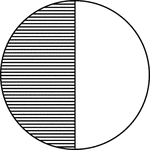### Circle, 1/2 Fraction

Illustration of a circle divided in half.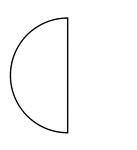### Half of a Fraction Pie

One half of a circle (vertical left).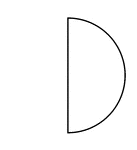### Half of a Fraction Pie

One half of a circle (vertical right).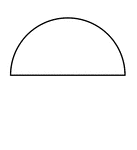### Half of a Fraction Pie

One half of a circle (horizontal top).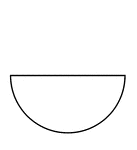### Half of a Fraction Pie

One half of a circle (horizontal bottom).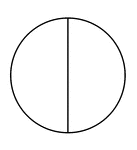### Two Halves of a Fraction Pie

A circle divided in half vertically.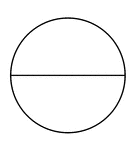### Two Halves of a Fraction Pie

A circle divided in half horizontally.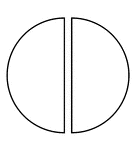### Two Halves of a Fraction Pie

A circle divided in half vertically separated.### Two Halves of a Fraction Pie

A circle divided in half horizontally separated.